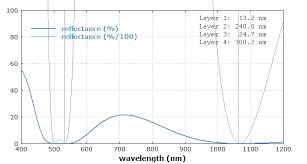# Anti-reflective lenses and interference

joshwarner
Homework Statement:
Anti-reflective coatings and interference
Relevant Equations:
While learning about wave interference in anti-reflective lenses, I came across something mentioning these lenses being only efficient for a certain wavelength. I know these lenses appear blue or purple because, when adding the layer, the reflectance for a wavelength over 600nm isn't worth 0 but is for a wavelength around 500nm. Why is that?Homework Helper
Gold Member
2022 Award
How do you think anti reflectance works?

joshwarner
How do you think anti reflectance works?
To remove the reflection on the glasses, the additional layer creates a new reflection of the light in order for the two waves reflected to interfere destructively when having a phase difference of an odd multiple of π. So using the distance traveled by the two waves, we get, at normal incidence, with w being the width of the coating layer : (k+1/2)λ=2nw+λ/2. However I still don't see how this explains the reflectance being worth 0 for λ=500nm.

Homework Helper
Gold Member
2022 Award
To remove the reflection on the glasses, the additional layer creates a new reflection of the light in order for the two waves reflected to interfere destructively when having a phase difference of an odd multiple of π. So using the distance traveled by the two waves, we get, at normal incidence, with w being the width of the coating layer : (k+1/2)λ=2nw+λ/2. However I still don't see how this explains the reflectance being worth 0 for λ=500nm.
Now I am not sure what your question is.
In post #1, I thought you were asking why the reflectance is reduced more at one wavelength than another. That should be evident from your equations in post #3; the ideal thickness of the coating depends on the wavelength.
But now you seem to be asking how it manages to achieve zero reflectance, even at the wavelength the thickness is optimised for.
Which is it?

joshwarner
Now I am not sure what your question is.
In post #1, I thought you were asking why the reflectance is reduced more at one wavelength than another. That should be evident from your equations in post #3; the ideal thickness of the coating depends on the wavelength.
But now you seem to be asking how it manages to achieve zero reflectance, even at the wavelength the thickness is optimised for.
Which is it?
Sorry for the confusion, I was asking why it was specifically for λ=500nm, I understand how it achieves a zero reflectance and why that only applies for a certain wavelength, but I didn't know it was for λ=500nm because of the thickness of the layer. Many thanks.